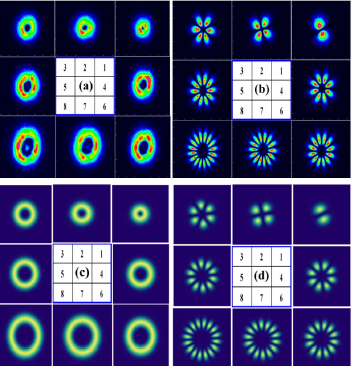Set Homepage | Favorite High Dimensional Quantum InterfaceHigh Dimensional Quantum Interface - Orbital Angular Momentum Frequency Conversion   Orbital angular momentum has an essential application in high-capacity optical communication, capture, and manipulation of small particles, imaging, precision measurement, and high-dimensional quantum information because of its particular spatial phase structure and infinite-dimensional Hilbert space. By using the orbital angular momentum conversion, the arbitrary transformation of orbital angular momentum beams of different wavelengths can be realized. In addition, the single-photon orbital angular momentum frequency transformation can realize the wavelength link of quantum orbit angular momentum quantum system with different wavelengths, and realize the orbital angular momentum information exchange in the quantum system of different wavelengths. The research is of great significance for the high dimensional quantum information based on orbital angular momentum.    Our group focused on the realization of the high-dimensional quantum interface, a key technology of high-capacity quantum network, to study the orbital angular momentum. The first time, the second-order nonlinear effect of the periodic nonlinear crystal was used to realize frequency upconversion — frequency conversion of the single photon, which lays the foundation for the realization of the high-dimensional quantum interface. In the PRA, OL, OE and other publications published a series of papers.-----------------------------------------------------------------------------Papers： Z.-Y. Zhou, Y. Li, D.-S. Ding, Y.-K. Jiang, W. Zhang, S. Shi, B.-S. Shi, and G.-C. Guo, Generation of light with controllable spatial patterns via the sum frequency in quasi-phase matching crystals, Scientific. Reports 4, 5650 (2014). Z.-Y. Zhou, D.-S. Ding, Y.-K. Jiang, Y. Li, S. Shi, X.-S. Wang, and B.-S. Shi, Orbital angular momentum light frequency conversion and interference with quasi-phase matching crystals, Optics Express 22, 20298 (2014). Z.-Y. Zhou, Y. Li, D.-S. Ding, Y.-K. Jiang, W. Zhang, S. Shi, B.-S. Shi, and G.-C. Guo, Highly efficient second harmonic generation of a light carrying orbital angular momentum in an external cavity, Optics Express 22, 23673 (2014). Y. Li, Z.-Y. Zhou, D.-S. Ding, and B.-S. Shi, Sum frequency generation with two orbital angular carrying laser beams, J. Opt. Soc. Am. B 32, 407(2015). Y. Li, Z.-Y. Zhou, D.-S. Ding, W. Zhang, S. Shi, B.-S. Shi, and G.-C. Guo, Orbital angular photonic quantum interface, arXiv:1410.7543 [quant-ph].